: 4 Dust transport : Two dimensional anelastic model : 2 Atmospheric model

# 3 Turbulent parameterization

## 3.1 Subgrid turbulent mixing parameterization

The turbulent diffusion coefficient is evaluated by the formula of Klemp and Wilhelmson (1978), where the turbulent diffusion is proportional to square root of turbulent kinetic energy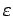. The value of turbulent diffusion coefficient for heat is equal to that for momentum. The diagnostic equation of turbulent kinetic energy is as follows.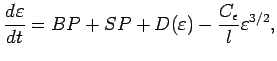(10)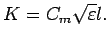(11)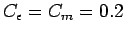.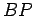and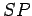are generation terms of the turbulent kinetic energy associated with buoyancy force and wind shear, respectively.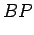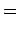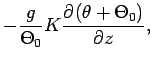(12)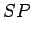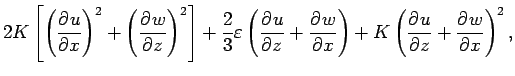(13)

where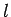is the mixing length which is the smaller value of either vertical grid interval or altitude.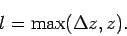The last term in left hand side of (10) represents the dissipation rate of turbulent kinetic energy. By using this term,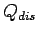in equation (5) is given as follows.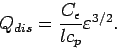(14)

## 3.2 Surface flux parameterization

The surface momentum and heat fluxes are given by the bulk formulae, where the bulk coefficients depend on static stability and vertical wind shear (Louis, 1979). The bulk coefficient for heat transport has same value of that for momentum.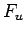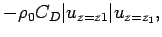(15)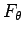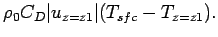(16)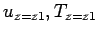are the horizontal wind and temperature at the lowest level of the model atmosphere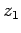.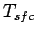is the surface temperature. The bulk coefficient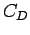is calculated as follows.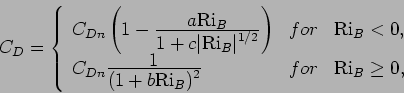(17)

where,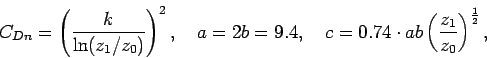(18)

and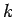is the Karman constant (= 0.35),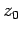is the roughness length.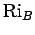is the bulk Richardson number, which is given as follow.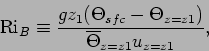(19)

where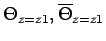and are the potential temperature and that of horizontal mean value at the lowest level of model atmosphere.

## Parameters

 Parameters Standard values Note0.351 cm Sutton et al., (1978): 4 Dust transport : Two dimensional anelastic model : 2 Atmospheric model
Odaka Masatsugu $BJ?@.(B19$BG/(B4$B7n(B25$BF|(B